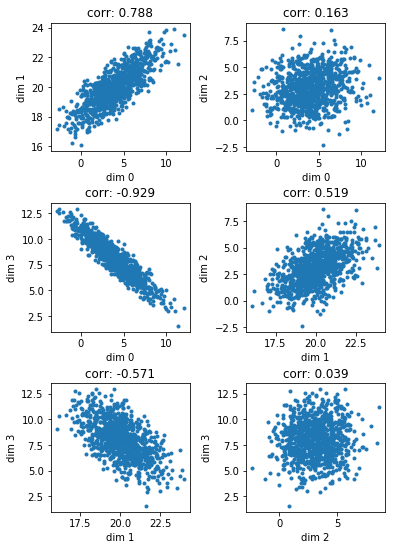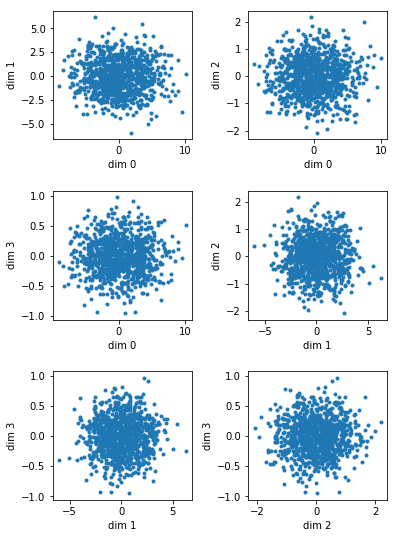The Components of Principal Component Analysis: A Python Tutorial

I recently ran a data science training course on the topic of principal component analysis and dimension reduction. This course was less about the intimate mathematical details, but rather on understanding the various outputs that are available when running PCA. In other words, my goal was to make sure that followers of this tutorial can see what terms like “explained_variance_” and “explained_variance_ratio_” and “components_” mean when they probe the PCA object. It shouldn’t be a mystery and it should be something that anyone can recreate “by hand”.

My training sessions tend to be fluid and no one session is the same as any other. Thus, this write-up is more a “paraphrasing” rather than an exact recount of events.

In this session, I created a four-dimensional data set with random pairwise covariances. Then I showed how to de-correlate this data with PCA and how eigenvectors and eigenvalues relate to the typical vocabulary that is encountered when reporting output of PCA.

In :
import math
import random
import itertools

In :
import pandas
import numpy
import scipy
import scipy.stats
import scipy.linalg
import sklearn
import sklearn.linear_model
import sklearn.decomposition
import matplotlib
import matplotlib.pyplot as plt


generate matrix of random numbers and a diagonal matrix for standard deviations¶

In :
size = 4
randmatrix = numpy.matrix(numpy.random.random((size,size))*2-1)
sigmas = numpy.matrix(numpy.identity(size)*[3,10,.4,.09])


generate a random covariance matrix¶

In :
z = numpy.matrix(scipy.linalg.orth(randmatrix))
cov = z.T*sigmas*z


compute correlation matrix¶

In :
temp = numpy.matrix(numpy.identity(size)*numpy.diagonal(cov)**-0.5)
corr = temp*cov*temp
corr

Out:
matrix([[ 1.        ,  0.77867357,  0.16056078, -0.93235238],
[ 0.77867357,  1.        ,  0.53569675, -0.56494582],
[ 0.16056078,  0.53569675,  1.        ,  0.05647073],
[-0.93235238, -0.56494582,  0.05647073,  1.        ]])

generate multivariate normal random numbers with specified means and covariance matrix¶

In :
means = [4,20,3,8]
rvs = numpy.random.multivariate_normal(means,cov.tolist(),1000)


eyeball empirical correlation matrix against theoretical from above¶

In :
empirical_corr = numpy.corrcoef(rvs.T)
empirical_corr

Out:
array([[ 1.        ,  0.78763524,  0.1625596 , -0.92942116],
[ 0.78763524,  1.        ,  0.5186283 , -0.57120149],
[ 0.1625596 ,  0.5186283 ,  1.        ,  0.03936412],
[-0.92942116, -0.57120149,  0.03936412,  1.        ]])

plot all pairwise relationships and eyeball behavior against correlation matrix¶

In :
fig,ax = plt.subplots(nrows = 3, ncols = 2, figsize = (6,9), gridspec_kw = {'height_ratios':*3,'width_ratios':*2})
fig.subplots_adjust(wspace = 0.4, hspace = 0.4)
for i,(a,b) in enumerate(itertools.combinations([i for i in range(4)],2)):
row = i//2
col = i%2
ax[row][col].scatter(rvs[:,a],rvs[:,b],marker = '.')
ax[row][col].set_xlabel('dim {a}'.format(a = a))
ax[row][col].set_ylabel('dim {b}'.format(b = b))
ax[row][col].set_title('corr: {corr:.3f}'.format(corr = empirical_corr[a][b]))sklearn PCA, fit and transform data¶

In :
pca = sklearn.decomposition.PCA(n_components = size)
pca.fit(rvs)
transformedrvs = pca.transform(rvs)


pca.explained_variance = eigenvalues of covariance matrix¶

Note the use of $$\texttt{ddof = 0}$$. For reasons, I haven’t yet been able to decipher, $$\texttt{pca.explained_variance}$$ and $$\texttt{eigenvalues}$$ coincide when $$\texttt{ddof = 0}$$ and sometimes when $$\texttt{ddof = 1}$$ (sampling penalty). Both are given here for the reader’s benefit.

In :
eigenvalues, eigenvectors = scipy.linalg.eig(numpy.cov(rvs.T,ddof=0))
eigenvalues, pca.explained_variance_, scipy.linalg.eig(numpy.cov(rvs.T,ddof=1))

Out:
(array([ 9.93581660+0.j,  2.97729164+0.j,  0.09061845+0.j,  0.44502942+0.j]),
array([ 9.9358166 ,  2.97729164,  0.44502942,  0.09061845]),
array([ 9.94576236+0.j,  2.98027191+0.j,  0.09070916+0.j,  0.44547489+0.j]))

observed covariance matrix of transformed data is diagonal matrix of eigenvalues¶

In :
numpy.cov(transformedrvs.T,ddof=0), numpy.diagonal(numpy.cov(transformedrvs.T,ddof=0)), eigenvalues, pca.explained_variance_

Out:
(array([[  9.93581660e+00,  -5.71986902e-16,  -1.03295150e-15,
-5.53157520e-15],
[ -5.71986902e-16,   2.97729164e+00,  -2.22044605e-16,
-3.49054119e-16],
[ -1.03295150e-15,  -2.22044605e-16,   4.45029419e-01,
-1.06914477e-16],
[ -5.53157520e-15,  -3.49054119e-16,  -1.06914477e-16,
9.06184532e-02]]),
array([ 9.9358166 ,  2.97729164,  0.44502942,  0.09061845]),
array([ 9.93581660+0.j,  2.97729164+0.j,  0.09061845+0.j,  0.44502942+0.j]),
array([ 9.9358166 ,  2.97729164,  0.44502942,  0.09061845]))

pca.components_ = eigenvectors of covariance matrix (up to sign change)¶

In :
eigenvectors.T, pca.components_

Out:
(array([[-0.76589999, -0.32089662, -0.1036887 ,  0.54742235],
[-0.04895795,  0.32138149,  0.90000225,  0.29036703],
[-0.62786518,  0.51622025, -0.03082423, -0.58168018],
[ 0.12955941,  0.72612393, -0.4222493 ,  0.52693826]]),
array([[ 0.76589999,  0.32089662,  0.1036887 , -0.54742235],
[-0.04895795,  0.32138149,  0.90000225,  0.29036703],
[ 0.12955941,  0.72612393, -0.4222493 ,  0.52693826],
[ 0.62786518, -0.51622025,  0.03082423,  0.58168018]]))

verify eigenvector matrix is orthogonal $$(Q^{T}Q = I)$$¶

In :
numpy.matrix(eigenvectors.T)*numpy.matrix(eigenvectors)

Out:
matrix([[  1.00000000e+00,   3.01599818e-16,   4.97036197e-17,
4.14905780e-16],
[  3.01599818e-16,   1.00000000e+00,   1.35607995e-16,
6.61439361e-17],
[  4.97036197e-17,   1.35607995e-16,   1.00000000e+00,
-1.83704831e-15],
[  4.14905780e-16,   6.61439361e-17,  -1.83704831e-15,
1.00000000e+00]])

pca.explained_variance_ratio_ for component $$i$$ is $$\frac{\lambda_{i}}{\sum_{i}\lambda_{i}}$$¶

In :
eigenvalues/eigenvalues.sum(), pca.explained_variance_ratio_, pca.explained_variance_/pca.explained_variance_.sum()

Out:
(array([ 0.73879075+0.j,  0.22138045+0.j,  0.00673805+0.j,  0.03309075+0.j]),
array([ 0.73879075,  0.22138045,  0.03309075,  0.00673805]),
array([ 0.73879075,  0.22138045,  0.03309075,  0.00673805]))

principal components are uncorrelated¶

In :
numpy.corrcoef(transformedrvs.T)

Out:
array([[  1.00000000e+00,  -1.05165578e-16,  -4.91228762e-16,
-5.82959945e-15],
[ -1.05165578e-16,   1.00000000e+00,  -1.92901310e-16,
-6.72006937e-16],
[ -4.91228762e-16,  -1.92901310e-16,   1.00000000e+00,
-5.32394835e-16],
[ -5.82959945e-15,  -6.72006937e-16,  -5.32394835e-16,
1.00000000e+00]])

verify that variance of transformed data equals the eigenvalues of the covariance matrix¶

In :
transformedrvs.std(axis = 0, ddof = 0)**2, eigenvalues, pca.explained_variance_

Out:
(array([ 9.9358166 ,  2.97729164,  0.44502942,  0.09061845]),
array([ 9.93581660+0.j,  2.97729164+0.j,  0.09061845+0.j,  0.44502942+0.j]),
array([ 9.9358166 ,  2.97729164,  0.44502942,  0.09061845]))

visually verify that transformed data is pairwise uncorrelated¶

In :
fig,ax = plt.subplots(nrows = 3, ncols = 2, figsize = (6,9), gridspec_kw = {'height_ratios':*3,'width_ratios':*2})
fig.subplots_adjust(wspace = 0.4, hspace = 0.4)
for i,(a,b) in enumerate(itertools.combinations([i for i in range(size)],2)):
row = i//2
col = i%2
ax[row][col].scatter(transformedrvs[:,a],transformedrvs[:,b],marker = '.')
ax[row][col].set_xlabel('dim {a}'.format(a = a))
ax[row][col].set_ylabel('dim {b}'.format(b = b))Notice in the above image of the six plots, the range of dimension 0 is the greatest, followed by dimension 1, then dimension 2, and finally dimension3. This should make sense since dimension 0 is the first principal component and thus explains the greatest variance in the data. Then each subsequent principal component explains a smaller amount of the variation. We can see from the explained_variance_ratio_ that the first two principal components explain roughly 96% of the variation in the data. So from a dimension reduction standpoint we can consider the first two principal components.

I hope this article helped you see how eigenvectors and eigenvalues fit in to the principal component analysis problem.

A request!

Thank you for reading! I want to keep in touch with my readers. If you are interested, please have a look here.

Do you enjoy this blog?
Consider supporting with a contribution!